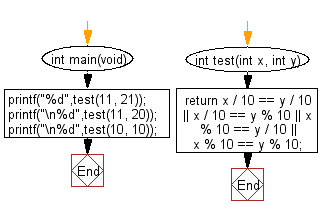﻿ C : Two integers in the range 10..99 have a digit in them# C Exercises: Check two given integers, each in the range 10..99. Return true if a digit appears in both numbers, such as the 3 in 13 and 33

## C-programming basic algorithm: Exercise-28 with Solution

Write a C program to check two given integers, each in the range 10..99. Return true if a digit appears in both numbers, such as the 3 in 13 and 33.

C Code:

``````#include <stdio.h>
#include <stdlib.h>

int main(void){
printf("%d",test(11, 21));
printf("\n%d",test(11, 20));
printf("\n%d",test(10, 10));
}
int test(int x, int y)
{
return x / 10 == y / 10 || x / 10 == y % 10 || x % 10 == y / 10 || x % 10 == y % 10;
}

``````

Sample Output:

```1
0
1
```

Pictorial Presentation:Flowchart:C Programming Code Editor:

What is the difficulty level of this exercise?

Test your Programming skills with w3resource's quiz.

﻿

## C Programming: Tips of the Day

Why doesn't a+++++b work?

printf("%d",a+++++b); is interpreted as (a++)++ + b according to the Maximal Munch Rule!.

++ (postfix) doesn't evaluate to an lvalue but it requires its operand to be an lvalue.

! 6.4/4 says the next preprocessing token is the longest sequence of characters that could constitute a preprocessing token"

Ref : https://bit.ly/3fdldUT